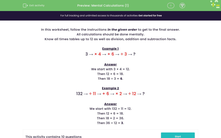# Mental Calculations (1)

In this worksheet, students perform mental calculations with mixed operations.Key stage:  KS 2

Curriculum topic:   Number: Addition, Subtraction, Multiplication and Division

Curriculum subtopic:   Perform Mental Calculations

Difficulty level:#### Worksheet Overview

In this worksheet, follow the instructions in the given order to get to the final answer.

All calculations should be done mentally.

Know all times tables up to 12 as well as division, addition and subtraction facts.

Example 1

3 → × 4+ 6÷ 3 → ?

Then 12 + 6 = 18.

Then 18 ÷ 3 = 6.

Example 2

132 → ÷ 11+ 6× 2÷ 12 → ?

Then 12 + 6 = 18.

Then 18 × 2 = 36.

Then 36 ÷ 12 = 3.

### What is EdPlace?

We're your National Curriculum aligned online education content provider helping each child succeed in English, maths and science from year 1 to GCSE. With an EdPlace account you’ll be able to track and measure progress, helping each child achieve their best. We build confidence and attainment by personalising each child’s learning at a level that suits them.

Get started